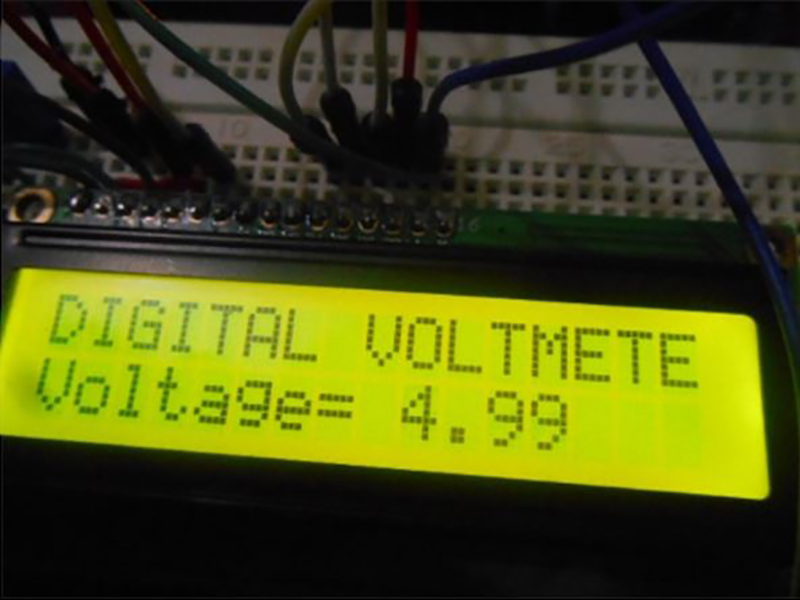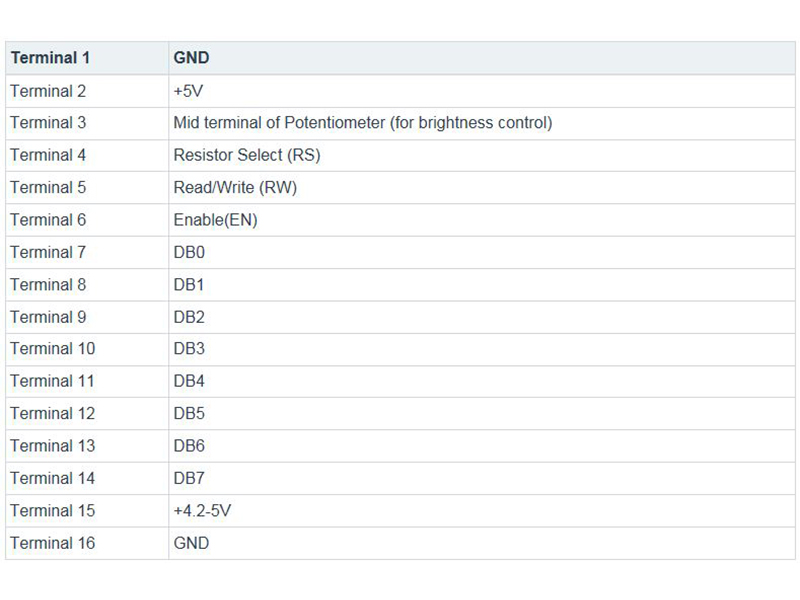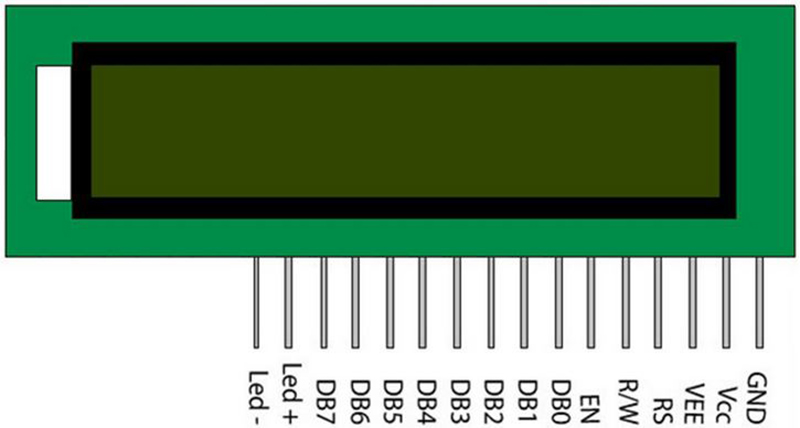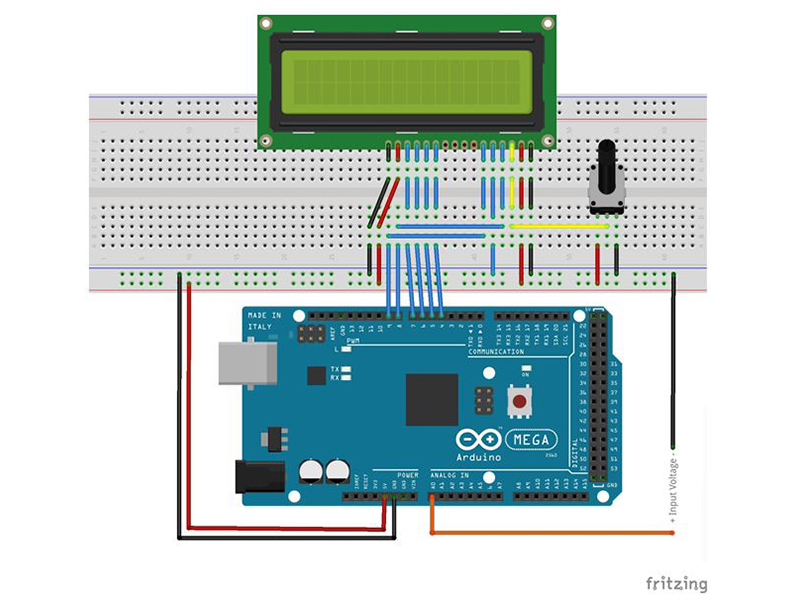# How to Make a Simple Digital Voltmeter with an ArduinoIt's easy to make a digital voltmeter. All that’s needed is an Arduino and a 16x2 liquid crystal display (LCD).

Using an Arduino to measure voltages is relatively simple. Inside the Arduino, there are multiple analog input pins connecting to an analog-to-digital converter (ADC). The Arduino ADC is a ten-bit converter, and the output value ranges from 0 to 1023. We will obtain this value using the analogRead() function. If you know the reference voltage—in this case we will use 5 V—you can easily calculate the voltage present at the analog input.

To display the measured voltage, we use a liquid crystal display (LCD) with two lines of 16 characters. LCDs are often used to display data from devices such as calculators, microwave ovens, and multiple other electrical appliances.

This project will also help you measure voltages above the reference voltage using a voltage divider.

Experiment 1

In this experiment, we will make digital voltmeter that measures up to 5V using a 16x2 LCD and an Arduino board.

Hardware Required

Wiring Diagram

The 16x2 LCD in this experiment has a sum of 16 pins. As seen in the table below, eight of the pins are data lines (pins 7-14), two are for ground and power (pins 1 and 16), three control the operation of the LCD (pins 4-6), and one adjusts the LCD screen brightness (pin 3). The remaining two pins (15 and 16) help power the backlight.Refer to the diagram below to see how to the Arduino with the LCD. Notice the potentiometer is connected to the 5V source and GND and the middle terminal is connected to pin 3 of LCD. Rotating this pot changes the LCD’s brightness. Four data pins DB4-DB7 are connected to Arduino pins 4-7. Enable connects to pin 9 of the Arduino, and RS connects to pin 8 of the Arduino. RW connects to ground. The backlight LED connects to 5V and ground. The table below shows the pin connections:

DB4 ----->pin4

DB5 ----->pin5

DB6 ----->pin6

DB7 ----->pin

7RS   ----->pin

8EN   ----->pin9Code

The program below uses the LiquidCrystal library. This library houses each of the functions needed to write to the LCD.

Using the analog input, the loop reads the analog value. The reference voltage is 5 V, so it multiples that value by 5. Afterward, it then divides by 1024 to calculate the actual voltage value. Once the voltage has been calculated, the value is written to the LCD.

The following photo shows a typical display.

Copy Code
`                    #include "LiquidCrystal.h"LiquidCrystal lcd(8, 9, 4, 5, 6, 7);float input_voltage = 0.0;float temp=0.0;void setup(){   Serial.begin(9600);     //  opens serial port, sets data rate to 9600 bps   lcd.begin(16, 2);       //// set up the LCD's number of columns and rows:    lcd.print("DIGITAL VOLTMETER");}void loop(){//Conversion formula for voltage      int analog_value = analogRead(A0);   input_voltage = (analog_value * 5.0) / 1024.0;       if (input_voltage < 0.1)    {     input_voltage=0.0;   }     Serial.print("v= ");    Serial.println(input_voltage);    lcd.setCursor(0, 1);    lcd.print("Voltage= ");    lcd.print(input_voltage);    delay(300);}`Experiment 2

In order to measure voltages higher than the 5 V reference voltage, you need to divide the input voltage. Then the voltage actually input to the Arduino is 5 V or less. in this experiment, we will use a 10 kohm, along with a 90.9 kohm resistor, to create a 10:1 divider. This will allow us to measure voltages up to 50 V.

Hardware Required

Wiring Diagram

The circuit for this experiment is the same as Experiment #1. However, now we have a voltage divider, made up of a 90.9 kohm resistor and a 10 kohm resistor that connects to the input. See the following diagram.Program

The program for this experiment is nearly the same as for Experiment #1. But, now we have to divide the voltage calculation by the ratio R2/(R1 + R2). In this case, it is 10,000/(90,900 + 10,000) ? 0.1.

Copy Code
`#include "LiquidCrystal.h"LiquidCrystal lcd(8, 9, 4, 5, 6, 7);float input_voltage = 0.0;float temp=0.0;float r1=90900.0;float r2=10000.0;void setup(){   Serial.begin(9600);     //  opens serial port, sets data rate to 9600 bps   lcd.begin(16, 2);       //// set up the LCD's number of columns and rows:    lcd.print("DIGITAL VOLTMETER");}void loop() { //Conversion formula    int analog_value = analogRead(A0);     temp = (analog_value * 5.0) / 1024.0;   input_voltage = temp / (r2/(r1+r2));        if (input_voltage < 0.1)   {      input_voltage=0.0;    }     Serial.print("v= ");    Serial.println(input_voltage);    lcd.setCursor(0, 1);lcd.print("Voltage= ");lcd.print(input_voltage);delay(300); }`

Videos

## Key Parts and Components

Add all Digi-Key Parts to Cart
• 1050-1018-ND
• 67-1779-ND
• 3310C-001-502L-ND
• 438-1046-ND
• 1528-2185-ND
• PPC90.9KXCT-ND
• PPC10.0KXCT-ND
• 3310C-001-502L-ND